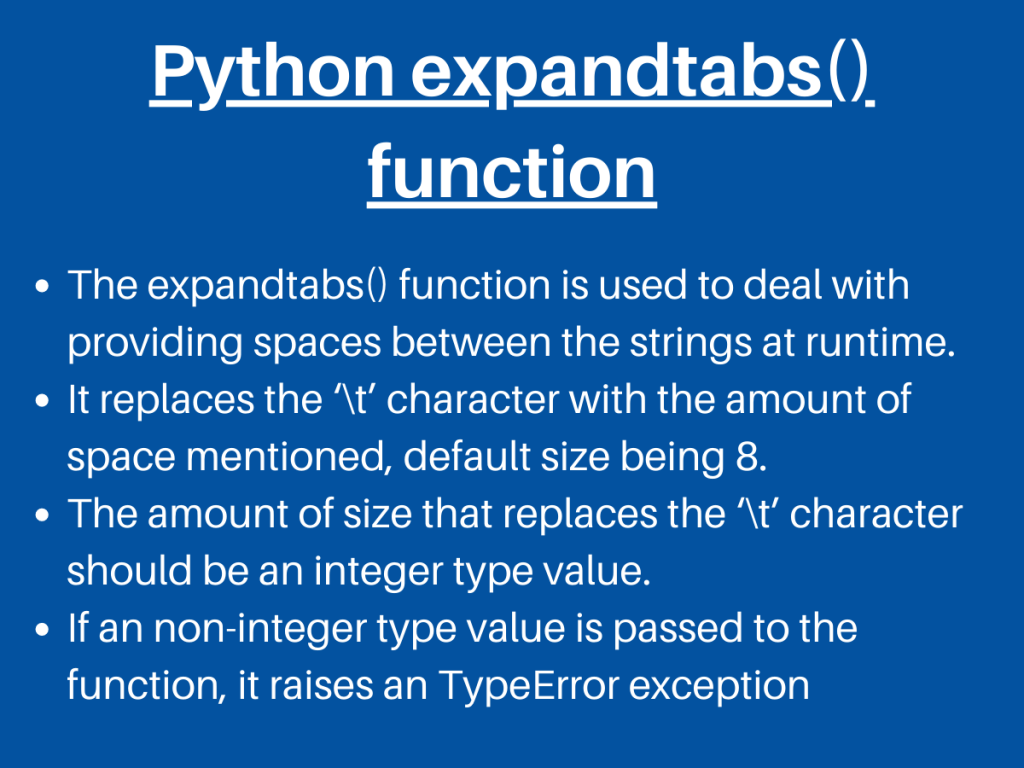# Understanding Python String expandtabs() FunctionPython has got an efficient way to deal with the handling of white spaces between the string. Let’s learn about the Python String expandtabs() method in this article.

## Working of Python String expandtabs() method

As mentioned above, Python String has got in-built expandtabs() method to handle the spaces between the strings.

The Python String `expandtabs()` function basically substitutes and expands the ‘\t’ character present in between the string with the amount of white space provided by the user as an argument.

Syntax:

```string.expandtabs(size)
```
• `size(optional)`: This parameter specifies the size in terms of space, that the user needs between the strings. The default size is 8.

Example 1:

```inp_str = "JournalDev\tprovides\ttutorials\ton\tPython\tand\tJava."
#print(inp_str)
res = inp_str.expandtabs()
print(res)
```

In the above example, the Python string expandtabs() function is used without any parameter i.e the size. Thus the ‘\t’ is replaced by the default size i.e. 8.

Output:

```JournalDev      provides        tutorials       on      Python  and     Java.
```

Example 2:

```inp_str = "JournalDev\tprovides\ttutorials\ton\tPython\tand\tJava."
print("Original string:\n",inp_str)
res = inp_str.expandtabs(4)
print("String after using expandtabs:\n",res)
```

In the above snippet of code, size = 4 is passed as an argument to the Python string expandtabs() function. Thus, the ‘\t’ character in the entire string is replaced by spaces of size 4 units.

Output:

```Original string:
JournalDev	provides	tutorials	on	Python	and	Java.
String after using expandtabs:
JournalDev  provides    tutorials   on  Python  and Java.
```

Example 3:

```inp_str = "JournalDev\tprovides\ttutorials\ton\tPython\tand\tJava."
print("Original string:\n",inp_str)
res = inp_str.expandtabs(18)
print("String after using expandtabs:\n",res)
```

Output:

```Original string:
JournalDev	provides	tutorials	on	Python	and	Java.
String after using expandtabs:
JournalDev        provides          tutorials         on                Python            and               Java.
```

## Python expandtabs() – Errors and Exceptions

If we try to pass a non-integer type value such as floating-point value as an argument to the Python string expandtabs() function, it raises a `TypeError exception`.

Thus, we can understand that the expandtabs() function accepts only an integer type value as a parameter.

Example:

```inp_str = "JournalDev\tprovides\ttutorials\ton\tPython\tand\tJava."
res = inp_str.expandtabs(18.17)
print("String after using expandtabs:\n",res)
```

Output:

```TypeError                                 Traceback (most recent call last)
<ipython-input-15-f2418be436bf> in <module>
1 inp_str = "JournalDev\tprovides\ttutorials\ton\tPython\tand\tJava."
----> 2 res = inp_str.expandtabs(18.17)
3 print("String after using expandtabs:\n",res)

TypeError: integer argument expected, got float

```

## Python numpy.expandtabs() function

Python Numpy module has numpy.char.expandtabs() function that serves the same functionality as that of in-built expandtabs() function.

The `numpy.char.expandtabs()` function accepts an array as an argument and the size of spaces in case the user wishes to be more specific.

Syntax:

```numpy.char.expandtabs(array,size)
```
• `array`: It contains the elements on which the function has to perform.
• `size`(optional): Being an optional parameter, it specifies the size of the spaces to be provided by replacing the tab-space character i.e. ‘\t’.

Example 1:

```import numpy
inp_arr = numpy.array("JournalDev\tprovides\ttutorials\ton\tPython\tand\tJava.")
res = numpy.char.expandtabs(inp_arr)
print("String after using expandtabs:\n",res)
```

Output:

```Array of string after using expandtabs:
JournalDev      provides        tutorials       on      Python  and     Java.
```

Example 2:

```import numpy
inp_arr = numpy.array("JournalDev\tprovides\ttutorials\ton\tPython\tand\tJava.")
res = numpy.char.expandtabs(inp_arr,20)
print("Array of string after using expandtabs:\n",res)
```

Output:

```Array of string after using expandtabs:
JournalDev          provides            tutorials           on                  Python              and                 Java.
```

## Summary

• The Python string expandtabs() function is used to deal with providing spaces between the strings at runtime. It replaces the ‘\t’ character with the amount of space mentioned, default size being 8.
• The amount of size that replaces the ‘\t’ should be an integer type value.
• If a non-integer type value is passed to the function, it raises a TypeError exception.
• Thus, expandtabs() proves out to be an efficient technique to deal with adding spaces at runtime.

## Conclusion

Thus, in this article, we have understood the working of Python string expandtabs() function with Python Strings and NumPy arrays.

## References

• Python expandtabs — JournalDev